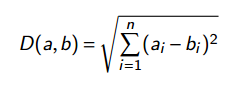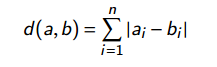STUDENT ML RESEARCHER

# K Nearest Neighbors AlgorithmKNN is one of the simplest supervised learning algorithm that makes predictions for a new data point. The KNN algorithm finds the nearest data points in the training dataset for prediction: its “nearest neighbors”. In the simplest form of KNN, the algorithm considers exactly one and only one of the nearest neighbors for the new entry. Predictions for the new data point are simply the known output. KNN is used both for regression and classification.

# KNN Analogy:

“A man is known by the company he keeps.” Just like in diagnosing a difficult case, a doctor could try to remember a similar case from the past. In KNN, the model representation is simply the training data. There is no model except storing the entire dataset and no learning is required. For new incoming data points, the prediction is made through the whole training sample for the K closest instances the neighbors and summing up the output variable for those K samples.

To find which of the K instances are most similar to a new input in the training dataset a distance measure is used. When the input is a real-valued variable, the well-known Euclidean distance measure is used.Hamming distance is also used between binary vectors, and Manhattan distance is used when the vector is real using the sum of their absolute difference.The K value can be found by parameter tuning. A good idea is to try different values of K and to check which one works best for a problem. The higher the K value, the smoother the decision boundary will be.

The KNN algorithm is also called the "lazy learning algorithm". This is because no learning is involved and the whole process occurs when a prediction is requested.

In KNNs, the infamous "curse of dimensionality" problem is a concern. The curse of dimensionality occurs when the number of inputs is quite large compared to the number of training samples. If there are n variables as input then it will be nth-dimensional. With the increase in dimensionality, the volume of the input space increases exponentially. The problem in high dimension space is all points will be far away from their neighbors.

The KNN algorithm works on the supposition that similar things are close to each other. The KNN is based on the idea of proximity, closeness, or distance with the mathematics of calculating distance between points.

How to choose the K value:

To select the value of K with the best fit for the data, the algorithm needs to run several times for different value of K. The machine learning engineer should choose the value of K that reduces the number of errors. Keep in mind that by decreasing the K value to 1, the model prediction will be less accurate. A lower value of K leads to noise and outliers in the model. When K is large, the prediction can potentially be more accurate due to averaging. In the case of majority voting, we mostly choose K as an odd number to eliminate the possibility of a tie.

1. The algorithm is simple and easy to implement.

2. No need to build or "train" a model.

3. The KNN algorithm is versatile can be used for classification as well as regression.